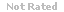Loading... Please wait...

A company's cost of capital is used as a tool in relation to which aspect of financial policy?

Price:
\$14.99

Product Description

1. A company's cost of capital is used as a tool in relation to which aspect of financial policy?
2. A company has a risk free rate of 3% and a risk premium of 6%. Its tax rate is 35%. What is the company's cost of debt?
3. A company has issued preferred stock that are valued at \$75 a share. The preferred dividend is \$5. The company's growth rate is 5%. What is the cost of the company's preferred stock?
4. A company issues common equity and has a beta of 1.5. The risk free return is 3% and the market return is 7%. What is the company's cost of common equity?
5. A company is thinking of issuing more common stock. Its stock's current market price is \$50 a share with a dividend of \$3 a share. The company has a 5% growth rate and a flotation cost of 10%. What is the company cost of new common stock?
6. A company has retained earnings of \$1.5 million and net income of \$8 million. What is the retention rate?
7. Which of the following interpretations of data related to a Security Market Line (SML) is correct?
8. In the capital asset pricing model (CAPM), you derive a stock’s:
9. In order to compute the statistical values in the capital asset pricing model (CAPM), you need information over time about:
10. Which of the following is NOT a way weighted average cost of capital (WACC) is used to analyze a company's financial activities?
11. Suppose that a company has total financing where 10% comes from bonds, 10% from a loan, and 80% from shareholders’ equity. The bonds pay on average a 10% interest rate, the loan has a 10% interest rate, and shareholders require a 10% return. The interest payment on the loan is tax deductible and the tax rate is 20%. What is the simple average cost of capital equal to?
12. Suppose that a company has total financing where 10% comes from bonds, 10% from a loan, and 80% from shareholders’ equity. The bonds pay on average a 10% interest rate, the loan has a 10% interest rate, and shareholders require a 10% return. What is the weighted average cost of capital (WACC) equal to?
13. Which of the following is a function of corporate capital budgeting?
14. Which of the following is the correct order of steps in a basic accounting flow?
15. You are analyzing two different investments and will present your findings to company executives. Both projects have cash flows that alternate between positive and negative. Which budgeting method should you use to evaluate the projects?
16. Which of the following is a correct definition of Net Present Value.
17. Which of the following reasons is a reason why a higher discount rate generally means a lower NPV?
18. A company is considering a project that has a discount rate of 5%. In the first year, it will have -\$100,000 in cash flows. In year 2, it will have cash flows of \$100,000, and in year 3 the project will generate \$200,000. What is the project's NPV?
19. Under the present value concept, a lottery winner would rather receive:
20. Which of the following criteria is NOT taken into consideration when analyzing a possible replacement project?

Customers Who Viewed This Product Also Viewed

• \$16.99
• \$12.99
• \$18.99
• \$12.99
• \$12.99

• \$14.99